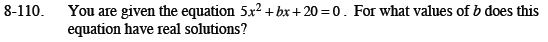Home > CCA2 > Chapter 8 > Lesson 8.2.3 > Problem8-110

8-110.

You are given the equation 5x2 + bx + 20 = 0. For what values of b does this equation have real solutions? Homework Help ✎The equation will have real solutions as long as the discriminant (the value under the radical) is greater than or equal to zero.

Solve the inequality b2 − 4ac ≥ 0 using the values for a and c in the given equation.